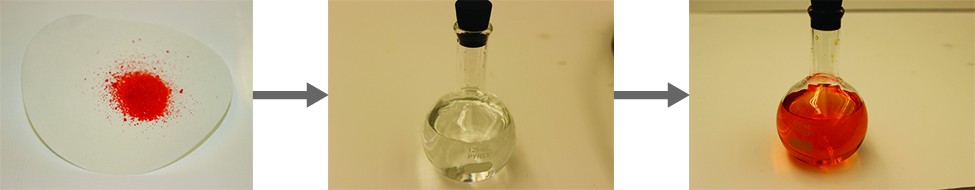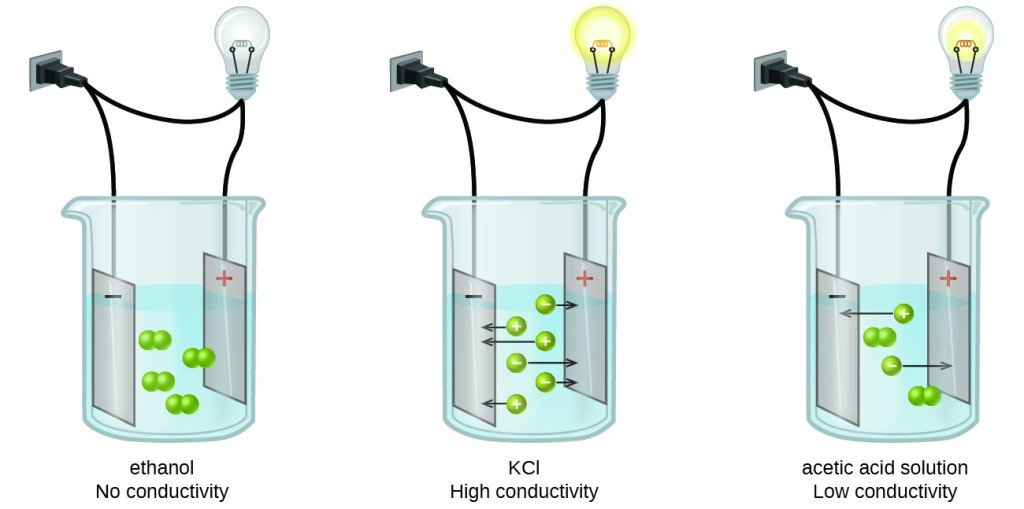## 9.1 Solutions

### Learning Objectives

By the end of this section, you will be able to:

• Describe the fundamental properties of solutions using correct terminology
• Classify solutions according to solubility
• Classify solutions according to conductivity

## Solutions

A solution is defined as homogeneous mixtures of two or more substances. Often, one component of a solution is present at a significantly greater concentration, in which case it is called the solvent. The other component of the solution present in relatively lesser concentrations are called solute. By major and minor we mean whichever component has the greater presence by mass or by moles. Sometimes this becomes confusing, especially with substances with very different molar masses. However, here we will confine the discussion to solutions for which the major component and the minor component are obvious.

Sugar is a covalent solid composed of sucrose molecules, C12H22O11. When this compound dissolves in water, its molecules become uniformly distributed among the molecules of water:

$\large{\text{C}}_{12}{\text{H}}_{22}{\text{O}}_{11}\left(s\right)\rightarrow{\text{C}}_{12}{\text{H}}_{22}{\text{O}}_{11}\left(aq\right)$

The subscript “aq” in the equation signifies that the sucrose molecules are solutes and are therefore individually dispersed throughout the aqueous solution (water is the solvent). Although sucrose molecules are heavier than water molecules, they remain dispersed throughout the solution; gravity does not cause them to “settle out” over time.

Potassium dichromate, K2Cr2O7, is an ionic compound composed of colorless potassium ions, K+, and orange dichromate ions, $\text{C}{\text{r}}_{2}{\text{O}}_{7}{}^{2-}\text{.}$ When a small amount of solid potassium chromate is added to water, the compound dissolves and dissociates to yield potassium ions and dichromate ions uniformly distributed throughout the mixture (Figure 1), as indicated in this equation:

$\large{\text{K}}_{2}\text{C}{\text{r}}_{2}{\text{O}}_{7}\left(s\right)\rightarrow 2{\text{ K}}^{+}\left(aq\right)+\text{C}{\text{r}}_{2}{\text{O}}_{7}\left(aq\right)$

As for the mixture of sugar and water, this mixture is also an aqueous solution. Its solutes, potassium and dichromate ions, remain individually dispersed among the solvent (water) molecules.Figure 1. When (left) potassium dichromate (K2Cr2O7) is mixed with (middle) water, it forms (right) a homogeneous orange solution. (credit: modifications of work by Mark Ott)

Visit the PhET Sugar and Salt Solutions virtual lab to view simulations of the dissolution of common covalent and ionic substances (sugar and salt) in water.

Water is used so often as a solvent that the word solution has come to imply an aqueous solution to many people. However, almost any gas, liquid, or solid can act as a solvent. Many alloys are solid solutions of one metal dissolved in another; for example, US five-cent coins contain nickel dissolved in copper. Air is a gaseous solution, a homogeneous mixture of nitrogen, oxygen, and several other gases. Oxygen (a gas), alcohol (a liquid), and sugar (a solid) all dissolve in water (a liquid) to form liquid solutions. Table 1 gives examples of several different solutions and the phases of the solutes and solvents.

Table 1. Different Types of Solutions
Solution Solute Solvent
air O2(g) N2(g)
soft drinks CO2(g) H2O(l)
rubbing alcohol H2O(l) C3H8O(l) (2-propanol)
saltwater NaCl(s) H2O(l)
brass Zn(s) Cu(s)

Solutions exhibit these defining traits:

• They are homogeneous; that is, after a solution is mixed, it has the same composition at all points throughout (its composition is uniform).
• The physical state of a solution—solid, liquid, or gas—is typically the same as that of the solvent, as demonstrated by the examples in Table 1.
• The components of a solution are dispersed on a molecular scale; that is, they consist of a mixture of separated molecules, atoms, and/or ions.
• The dissolved solute in a solution will not settle out or separate from the solvent.
• The composition of a solution, or the concentrations of its components, can be varied continuously, within limits.

### Example 1: Solutions

A solution is made by dissolving 1.00 g of sucrose (C12H22O11) in 100.0 g of liquid water. Identify the solvent and solute in the resulting solution.

A solution is made by dissolving 3.33 g of HCl(g) in 40.0 g of liquid methyl alcohol (CH3OH). Identify the solvent and solute in the resulting solution.

## Classifying Solutions According to Solubility

Imagine adding a small amount of salt to a glass of water, stirring until all the salt has dissolved, and then adding a bit more. You can repeat this process until the salt concentration of the solution reaches its natural limit. You can be certain that you have reached this limit because, no matter how long you stir the solution, undissolved salt remains. The concentration of salt in the solution at this point is known as its solubility.

The solubility of a solute in a particular solvent is the maximum concentration that may be achieved under given conditions when the dissolution process is at equilibrium. Referring to the example of salt in water:

$\large\text{NaCl}\left(s\right){\rightleftharpoons}{\text{ Na}}^{+}\left(aq\right)+{\text{Cl}}^{-}\left(aq\right)\text{.}$

When a solute’s concentration is equal to its solubility, the solution is said to be saturated with that solute. If the solute’s concentration is less than its solubility, the solution is said to be unsaturated. A solution that contains a relatively low concentration of solute is called dilute, and one with a relatively high concentration is called concentrated.

If we add more salt to a saturated solution of salt, we see it fall to the bottom and no more seems to dissolve. In fact, the added salt does dissolve, as represented by the forward direction of the dissolution equation. Accompanying this process, dissolved salt will precipitate, as depicted by the reverse direction of the equation. The system is said to be at equilibrium when these two reciprocal processes are occurring at equal rates, and so the amount of undissolved and dissolved salt remains constant. Support for the simultaneous occurrence of the dissolution and precipitation processes is provided by noting that the number and sizes of the undissolved salt crystals will change over time, though their combined mass will remain the same.

Use this PhET interactive simulation on Salts and Solubility to prepare various saturated solutions.

In some circumstances, it is possible to dissolve more than the maximum amount of a solute in a solution. Usually, this happens by heating the solvent, dissolving more solute than would normally dissolve at regular temperatures, and letting the solution cool down slowly and carefully. Such solutions are called supersaturated solutions and are not stable; given an opportunity (such as dropping a crystal of solute in the solution), the excess solute will precipitate from the solution.

Table 1. Solubilities of Some Ionic Compounds

Solute Solubility (g per 100 g of H2O at 25°C)
AgCl 0.00019
CaCO3 0.0006
KBr 70.7
NaCl 36.1
NaNO3 94.6

### Example 2: Classifying Solutions according to solubility

Sodium acetate has a solubility of 123.3 g/100 g of water  at 20 °C.  Is a solution containing 45.0 g of sodium acetate per 100 g of H2O is unsaturated, saturated, or supersaturated.

Sodium nitrate has a solubility of 94.6 g/100 g of water  at 20 °C. If 97.8 g of sodium nitrate is added to 100 g of water, the resulting solution will be unsaturated, saturated, or supersaturated?

Is a solution containing 45.0 g of sodium acetate per 100 g of H2O is unsaturated, saturated, or supersaturated.

## Classifying Solutions According to Conductivity

When some substances are dissolved in water, they undergo either a physical or a chemical change that yields ions in solution. These substances constitute an important class of compounds called electrolytes. Substances that do not yield ions when dissolved are called nonelectrolytes. If the physical or chemical process that generates the ions is essentially 100% efficient (all of the dissolved compound yields ions), then the substance is known as a strong electrolyte. If only a relatively small fraction of the dissolved substance undergoes the ion-producing process, it is called a weak electrolyte.

Substances may be identified as strong, weak, or nonelectrolytes by measuring the electrical conductance of an aqueous solution containing the substance. To conduct electricity, a substance must contain freely mobile, charged species. Most familiar is the conduction of electricity through metallic wires, in which case the mobile, charged entities are electrons. Solutions may also conduct electricity if they contain dissolved ions, with conductivity increasing as ion concentration increases. Applying a voltage to electrodes immersed in a solution permits assessment of the relative concentration of dissolved ions, either quantitatively, by measuring the electrical current flow, or qualitatively, by observing the brightness of a light bulb included in the circuit (Figure 1).Figure 1. Solutions of nonelectrolytes such as ethanol do not contain dissolved ions and cannot conduct electricity. Solutions of electrolytes contain ions that permit the passage of electricity. The conductivity of an electrolyte solution is related to the strength of the electrolyte.

Most ionic compounds we come across in this course will be strong electrolytes.  However, to truly know if an ionic compound dissociates in water we must rely on the solubility rules (Table 2).

Table 2. Solubility Rules

 These compounds generally dissolve in water (are soluble): Exceptions: All compounds of Li+, Na+, K+, Rb+, Cs+, and NH4+ None All compounds of NO3− and C2H3O2− None Compounds of Cl−, Br−, I− Ag+, Hg22+, Pb2+ Compounds of SO42 Hg22+, Pb2+, Sr2+, Ba2+ These compounds generally do not dissolve in water (are insoluble): Exceptions: Compounds of CO32− and PO43− Compounds of Li+, Na+, K+, Rb+, Cs+, and NH4+ Compounds of OH− Compounds of Li+, Na+, K+, Rb+, Cs+, NH4+, Sr2+, and Ba2+

### Key Takeaways

• Solutions are composed of a solvent (major component) and a solute (minor component).
• Concentration is the expression of the amount of solute in a given amount of solvent and can be described by several qualitative terms.
• Solubility is a specific amount of solute that can dissolve in a given amount of solvent.

### Exercises

1. Define solute and solvent.

2. Define saturated, unsaturated, and supersaturated.

3. A solution is prepared by combining 2.09 g of CO2 and 35.5 g of H2O. Identify the solute and solvent.

4. A solution is prepared by combining 10.3 g of Hg(ℓ) and 45.0 g of Ag(s). Identify the solute and solvent.

5. Use  Table 1 to decide if a solution containing 45.0 g of NaCl per 100 g of H2O is unsaturated, saturated, or supersaturated.

6. Use Table 1 to decide if a solution containing 0.000092 g of AgCl per 100 g of H2O is unsaturated, saturated, or supersaturated.

## Glossary

dissociation: physical process accompanying the dissolution of an ionic compound in which the compound’s constituent ions are solvated and dispersed throughout the solution

electrolyte: substance that produces ions when dissolved in water

nonelectrolyte: substance that does not produce ions when dissolved in water

saturated: of concentration equal to solubility; containing the maximum concentration of solute possible for a given temperature and pressure

solubility: maximum concentration of a solute that may be achieved at a given temperature and pressure

strong electrolyte: substance that dissociates or ionizes completely when dissolved in water

supersaturated: of concentration that exceeds solubility; a nonequilibrium state

unsaturated: of concentration less than solubility

weak electrolyte: substance that ionizes only partially when dissolved in water

1. If bubbles of gas are observed within the liquid, the mixture is not homogeneous and, thus, not a solution.